# Plot types#

Overview of many common plotting commands provided by Matplotlib.

See the gallery for more examples and the tutorials page for longer examples.

## Pairwise data#

Plots of pairwise $$(x, y)$$, tabular $$(var\_0, \cdots, var\_n)$$, and functional $$f(x)=y$$ data.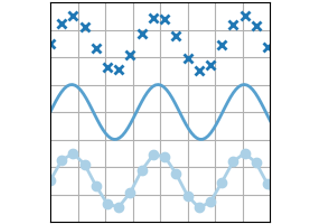plot(x, y)

plot(x, y)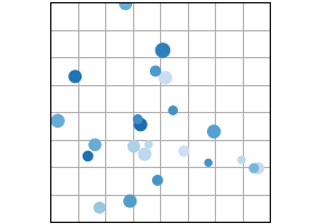scatter(x, y)

scatter(x, y)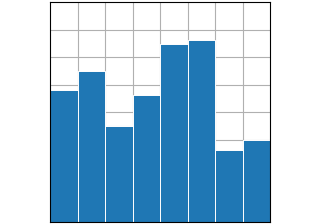bar(x, height)

bar(x, height)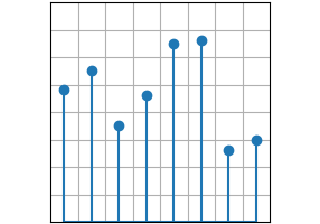stem(x, y)

stem(x, y)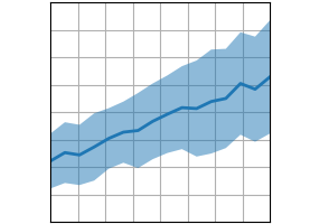fill_between(x, y1, y2)

fill_between(x, y1, y2)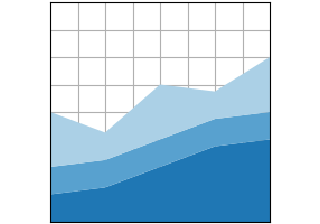stackplot(x, y)

stackplot(x, y)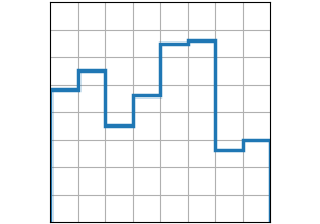stairs(values)

stairs(values)

## Statistical distributions#

Plots of the distribution of at least one variable in a dataset. Some of these methods also compute the distributions.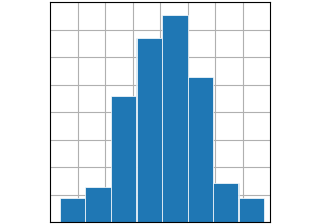hist(x)

hist(x)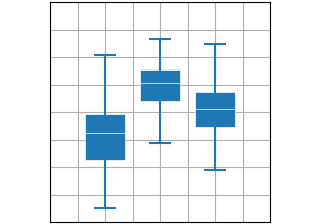boxplot(X)

boxplot(X)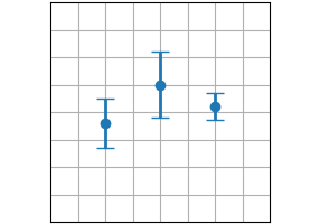errorbar(x, y, yerr, xerr)

errorbar(x, y, yerr, xerr)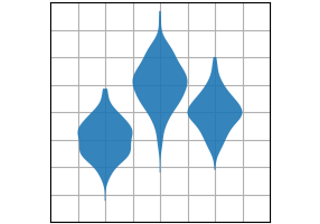violinplot(D)

violinplot(D)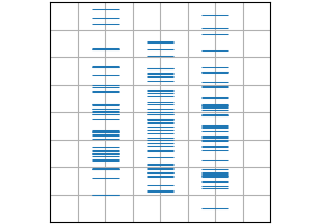eventplot(D)

eventplot(D)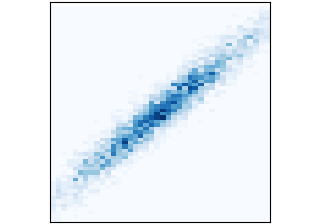hist2d(x, y)

hist2d(x, y)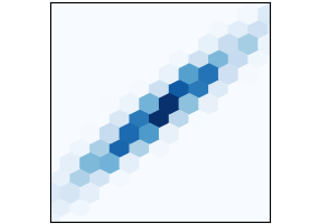hexbin(x, y, C)

hexbin(x, y, C)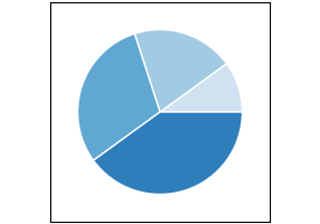pie(x)

pie(x)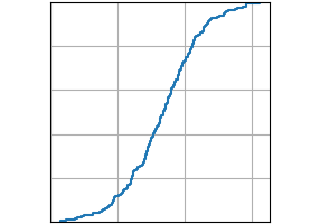ecdf(x)

ecdf(x)

## Gridded data:#

Plots of arrays and images $$Z_{i, j}$$ and fields $$U_{i, j}, V_{i, j}$$ on regular grids and corresponding coordinate grids $$X_{i,j}, Y_{i,j}$$.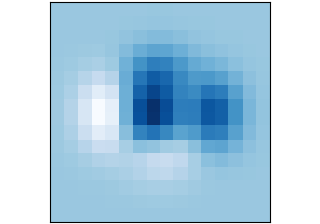imshow(Z)

imshow(Z)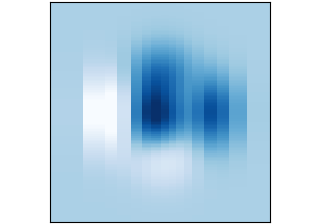pcolormesh(X, Y, Z)

pcolormesh(X, Y, Z)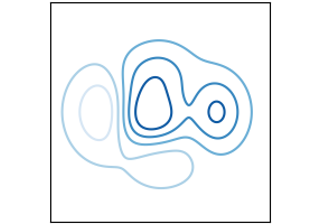contour(X, Y, Z)

contour(X, Y, Z)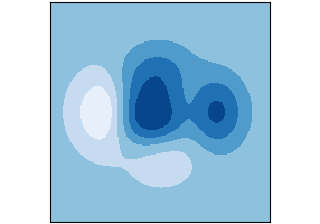contourf(X, Y, Z)

contourf(X, Y, Z)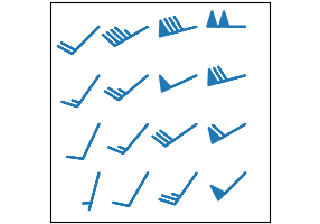barbs(X, Y, U, V)

barbs(X, Y, U, V)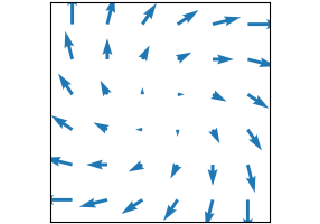quiver(X, Y, U, V)

quiver(X, Y, U, V)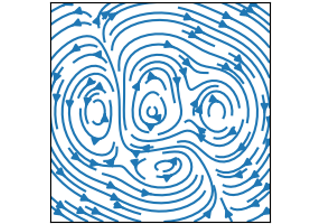streamplot(X, Y, U, V)

streamplot(X, Y, U, V)

## Irregularly gridded data#

Plots of data $$Z_{x, y}$$ on unstructured grids , unstructured coordinate grids $$(x, y)$$, and 2D functions $$f(x, y) = z$$.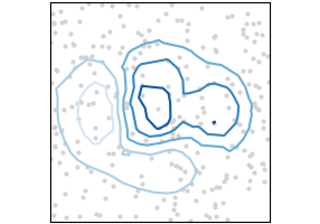tricontour(x, y, z)

tricontour(x, y, z)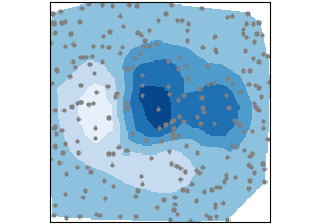tricontourf(x, y, z)

tricontourf(x, y, z)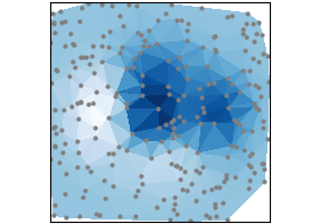tripcolor(x, y, z)

tripcolor(x, y, z)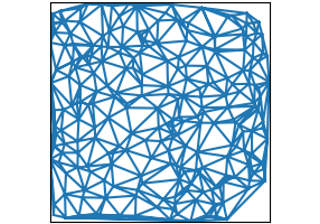triplot(x, y)

triplot(x, y)

## 3D and volumetric data#

Plots of three-dimensional $$(x,y,z)$$, surface $$f(x,y)=z$$, and volumetric $$V_{x, y, z}$$ data using the mpl_toolkits.mplot3d library.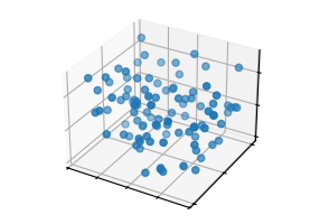scatter(xs, ys, zs)

scatter(xs, ys, zs)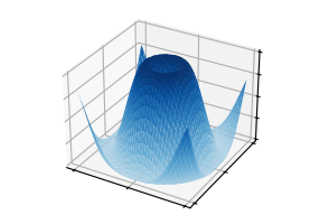plot_surface(X, Y, Z)

plot_surface(X, Y, Z)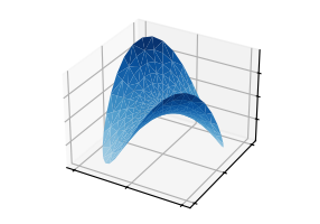plot_trisurf(x, y, z)

plot_trisurf(x, y, z)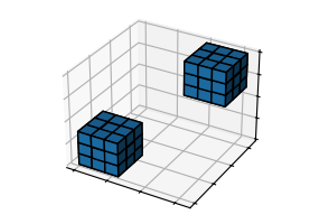voxels([x, y, z], filled)

voxels([x, y, z], filled)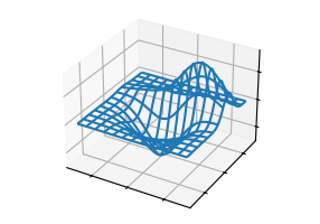plot_wireframe(X, Y, Z)

plot_wireframe(X, Y, Z)

Gallery generated by Sphinx-Gallery